# Rectangular Prism

Rectangular Prism
Go back to  'Shapes'

Prisms are solids with identical polygon ends and flat parallelogram sides.

The different types of prisms are triangular prisms, square prisms, rectangular prisms, pentagonal prisms, hexagonal prisms, etc.

A rectangular prism is a three-dimensional shape, having six faces, and all the faces of the prism are rectangles.

Both, the base and the top of a rectangular prism must be rectangles. Also, the lateral faces are rectangles.

Let us learn more about rectangular prism, right rectangular prism, cuboids, and cubes in the concept of Rectangular Prism.

Check-out the interactive simulation to calculate the volume of a rectangular prism. Try your hand at solving a few interesting practice questions at the end of the page.

## What is Rectangular Prism?

A rectangular prism is a three-dimensional shape, having six faces, and all the faces of the prism are rectangles.

Both, the base and the top of a rectangular prism must be rectangles. Also, the lateral faces are rectangles.

Examples

• Rectangular tissue box, school notebooks, and binders, laptops, cereal boxes, fish tanks, etc.
• Large structures such as cargo containers, buildings, storage sheds, and skyscrapers.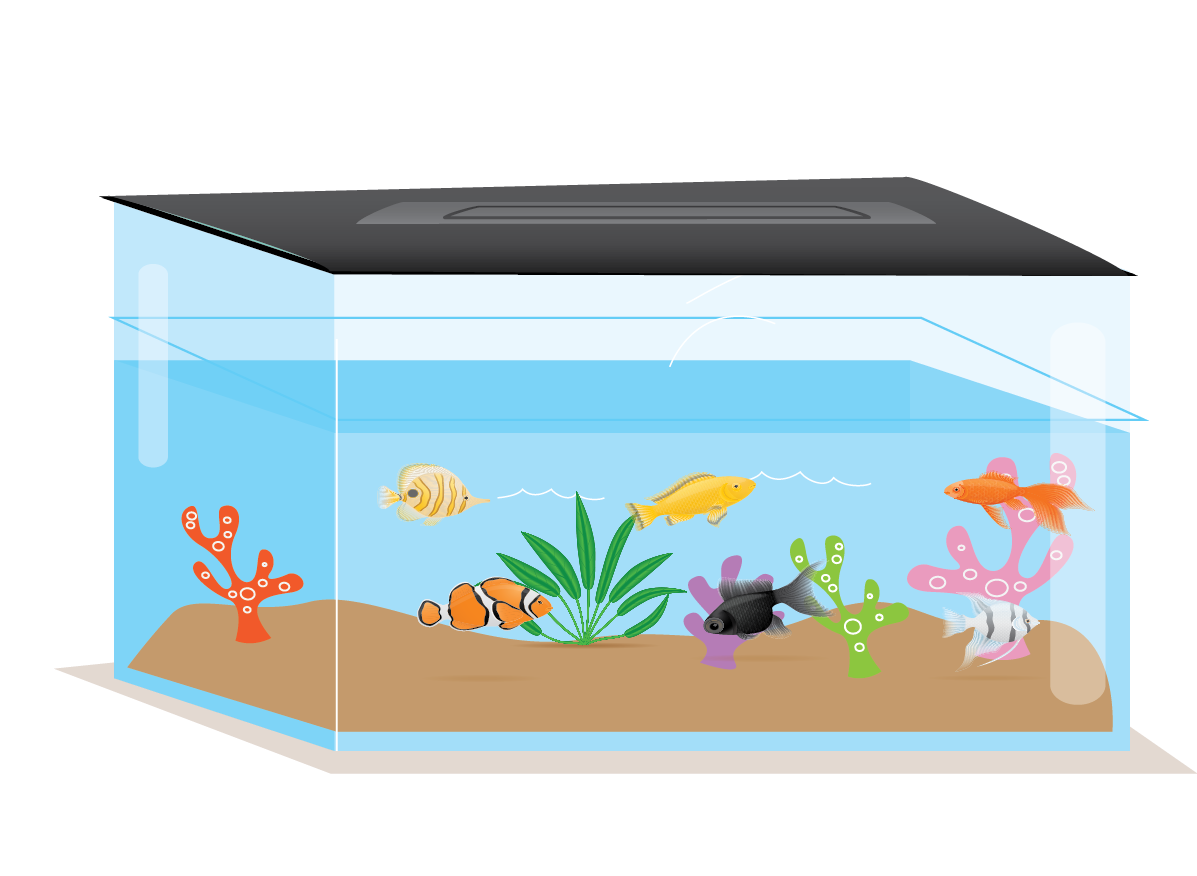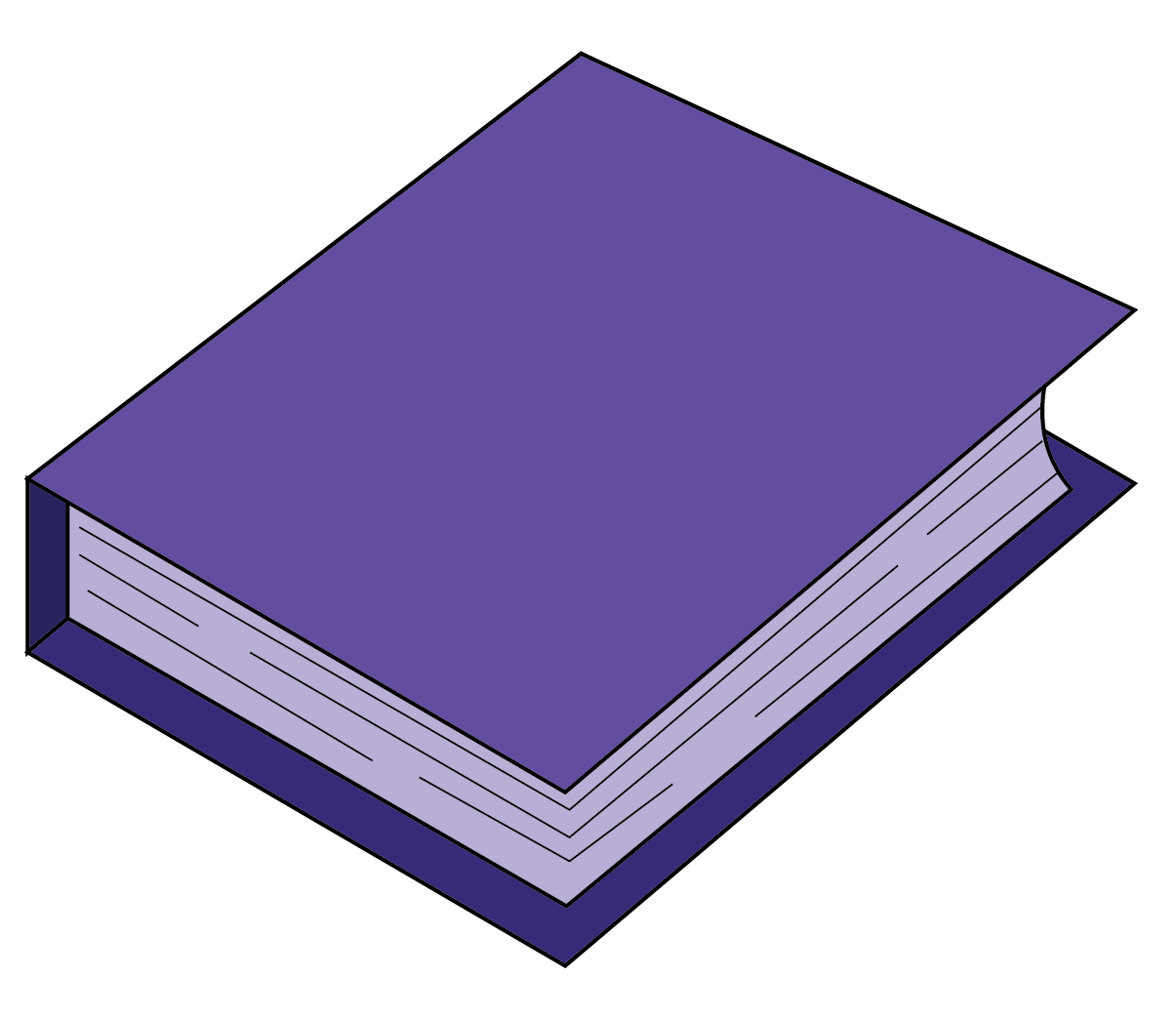### Right Rectangular Prism

In a right rectangular prism:

• The angles between the base and the sides are right angles.
• All its faces are rectangles.
• Each corner of the prism represents a right angle.
• Each base and top of the prism is congruent.Think Tank
1. Is cube a rectangular prism?
2. Can you determine the volume of a rectangular prism when the area of its base and height are given but length and width are not given as separate measures?

## Rectangular Prism Properties

• A rectangular prism has 6 faces(each of them being rectangular),12 sides, and 8 vertices.
• The pairs of opposite faces of a rectangular prism are equal.
• It has a rectangular cross-section, the same cross-section along the length.
• There are two types of rectangular prisms, namely right rectangular prism and non-right rectangular prisms.

## Rectangular Prism Formula

### Rectangular Prism Surface Area

Surface area of a rectangular prism = sum of surface area of six faces = $$lw+lw+wh+wh+lh+lh$$

$$= 2(lw+wh+lh)$$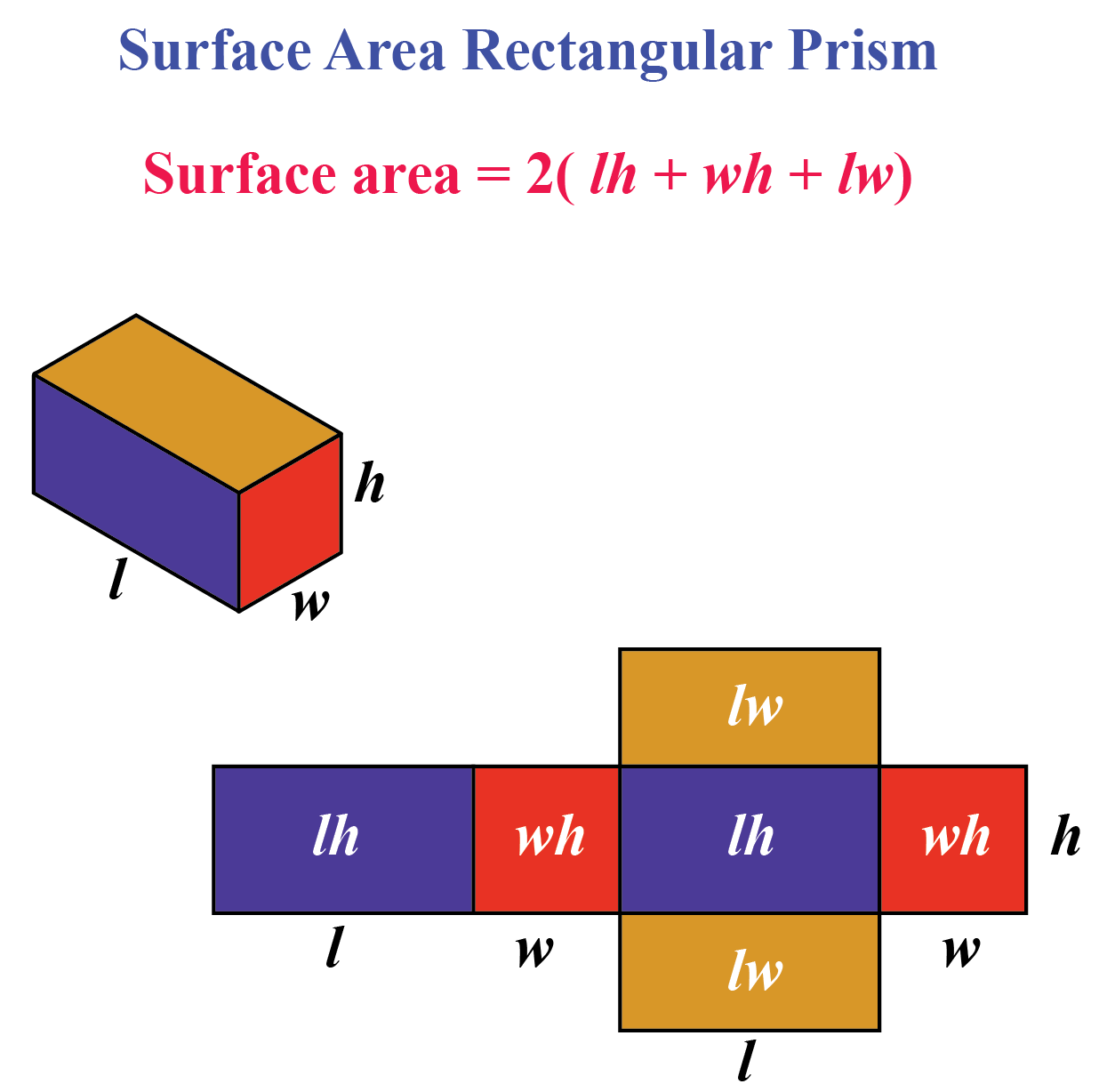### Rectangular Prism Volume

The volume of a Rectangular Prism (V) = l × w × h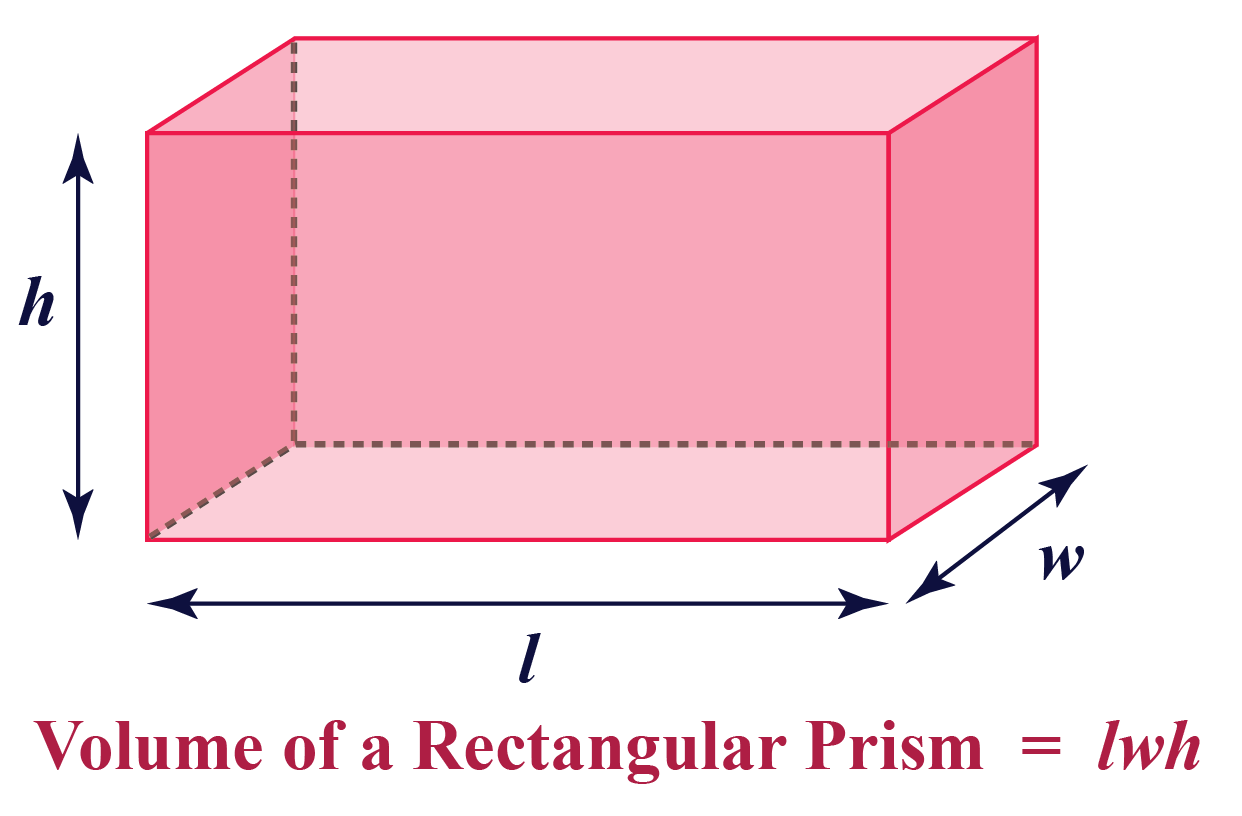Explore the simulation given below and calculate the volume of a rectangular prism:

## How do you find the Volume of Rectangular Prism?

Have a look at the image below to see how the volume of a rectangular prism can be calculated.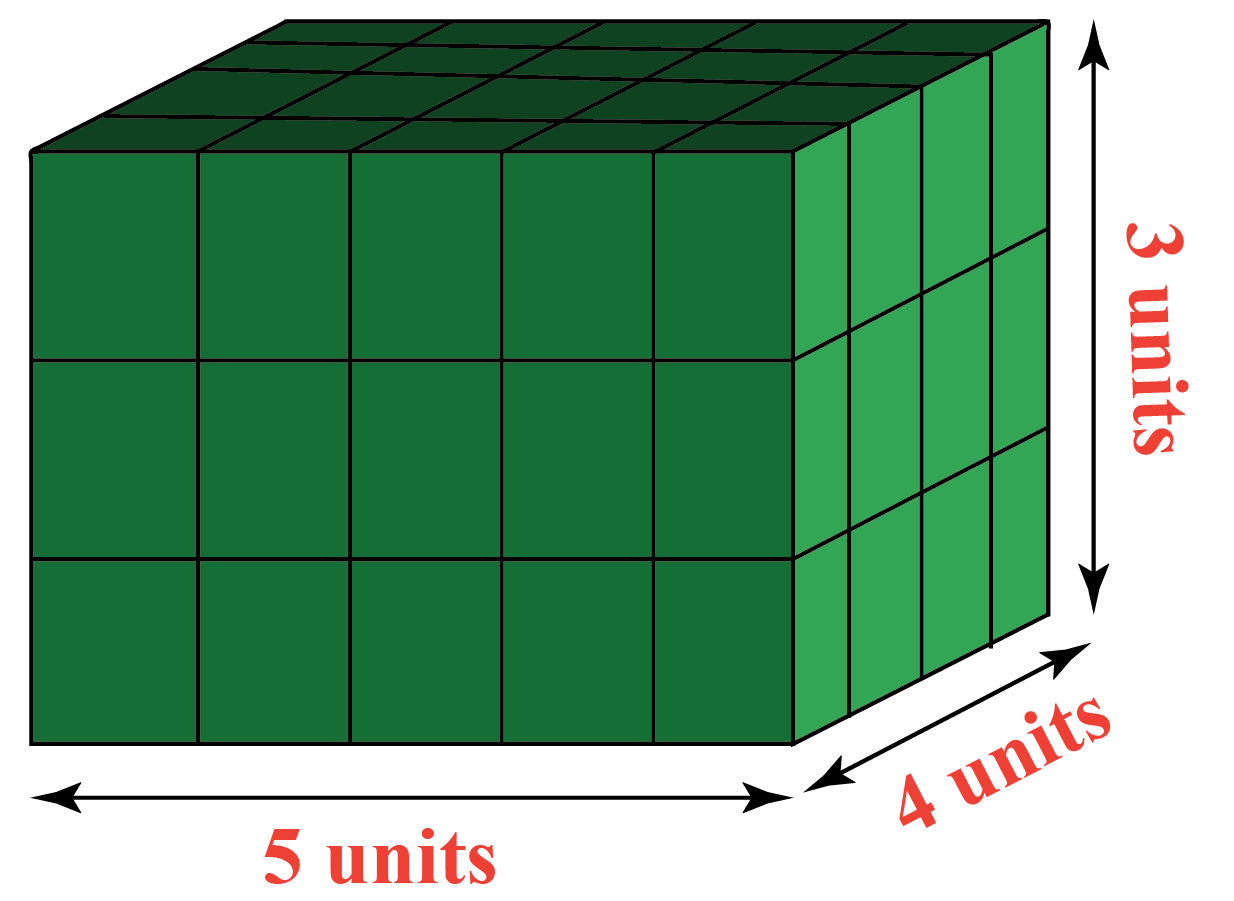How many cubic unit blocks are required to fill a rectangular prism of length 5 units, width 4 units, and height 3 units?

What is the volume of the rectangular prism?

5 blocks in each row.

4 rows in each layer.

3 layers in total.

Total number of blocks = 5 x 4 x 3 = 60

So the volume of rectangular prism = 60 cubic units.

Thus,

Volume of a Rectangular Prism (V) = l × w × h

More Important Topics
Numbers
Algebra
Geometry
Measurement
Money
Data
Trigonometry
Calculus